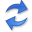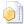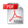Telling Causal From Confounded

Abstract. Given data over variables $$(X_1,...,X_n, Y)$$ we consider the problem of finding out whether $$X$$ jointly causes $$Y$$ or whether they are all confounded by an unobserved latent variable $$Z$$. To do so, we take an information-theoretic approach based on Kolmogorov complexity. In a nutshell, we follow the postulate that first encoding the true cause, and then the effects given that cause, results in a shorter description than any other encoding of the observed variables.

The ideal score is not computable, and hence we have to approximate it. We propose to do so using the Minimum Description Length (MDL) principle. We compare the MDL scores under the models where $$X$$ causes $$Y$$ and where there exists a latent variables $$Z$$ confounding both $$X$$ and $$Y$$ and show our scores are consistent. To find potential confounders we propose using latent factor modeling, in particular, probabilistic PCA (PPCA).

Empirical evaluation on both synthetic and real-world data shows that our method, CoCa, performs very well—even when the true generating process of the data is far from the assumptions made by the models we use. Moreover, it is robust as its accuracy goes hand in hand with its confidence.

## Implementationthe Python source code (February 2019) by David Kaltenpoth.

## Related PublicationsKaltenpoth, D & Vreeken, J We Are Not Your Real Parents: Telling Causal From Confounded by MDL. In: SIAM International Conference on Data Mining (SDM), SIAM, 2019. (22.9% acceptance rate)Kaltenpoth, D & Vreeken, J We Are Not Your Real Parents: Telling Causal From Confounded by MDL. Technical Report 1901.06950, arXiv, 2019.